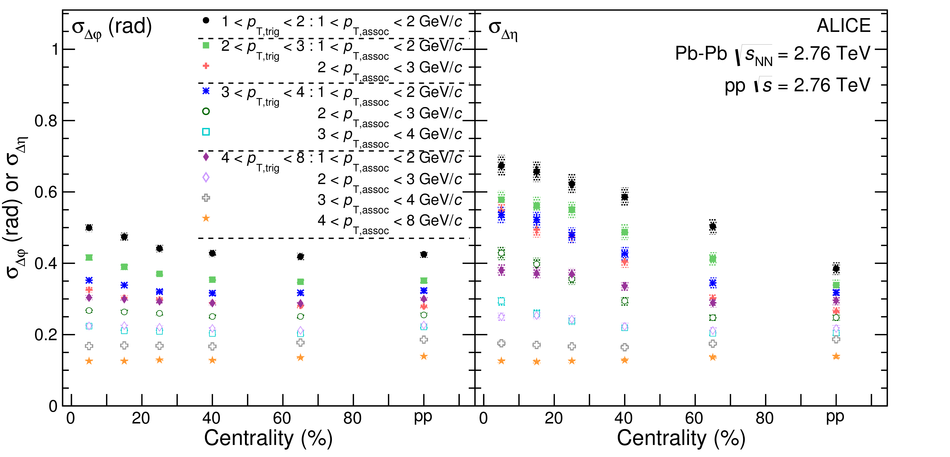# Figure 5

 Shape parameters $\sigma_{\Dphi}$ (left panel) and $\sigma_{\Deta}$ (right panel) as a function of centrality in different $\pt$ ranges for Pb--Pb collisions at \snn\ = \unit[2.76]{TeV} and pp collisions (right most points in each panel). Lines indicate statistical uncertainties (mostly smaller than the marker size), while boxes denote systematic uncertainties. The markers are placed at the centre of the centrality bins